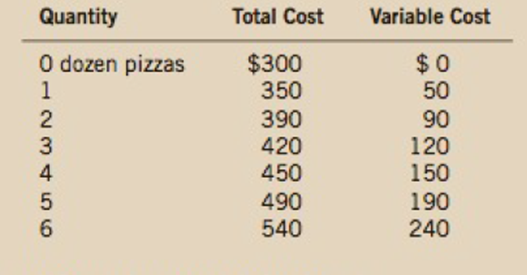Chapter 13, Problem 6PA

Chapter
Section
Textbook Problem

Consider the following cost information for a pizzeria:a. What is the pizzeria’s fixed cost?b. Construct a table in which you calculate the marginal cost per dozen pizzas using the information on total cost. Also, calculate the marginal cost per dozen pizzas using the information on variable cost. What is the relationship between these sets of numbers? Explain.

Subpart (a):

To determine
A fixed cost, marginal cost, and total cost.

Explanation

Table - 1 shows the cost information for a pizzeria:

Table - 1

 Quantity Total cost Variable cost 0 300 0 1 350 50 2 390...

Subpart (b):

To determine
A fixed cost, marginal cost, and total cost.

Still sussing out bartleby?

Check out a sample textbook solution.

See a sample solution

The Solution to Your Study Problems

Bartleby provides explanations to thousands of textbook problems written by our experts, many with advanced degrees!

Get Started

Why is productivity important?

Principles of Economics (MindTap Course List)

What is a loan amortization schedule, and what are some ways these schedules are used?

Fundamentals of Financial Management, Concise Edition (MindTap Course List)

Name the two types of ledgers.

Accounting Information Systems

If interest rates rise after a bond issue, what will happen to the bonds price and YTM? Does the time to maturi...

Fundamentals of Financial Management, Concise Edition (with Thomson ONE - Business School Edition, 1 term (6 months) Printed Access Card) (MindTap Course List)